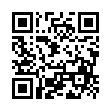# North Coast Synthesis Ltd.

## B minor fourth, add second, add sharp rootChoose audio sample set:
MSK 007 Leapfrog VCF (patch; see in online store)
MSK 009 Coiler VCF (patch; see in online store)

Equivalent chord symbols: Bm4+2+♭2, Bm4+2+♭9, D2+6+7+♯7, D2+6+7+♭1, C3+2+♯1+♯7, C3+2+♯1+♭1.

Notes in this chord: B, B♯, C♯, D, E. Integer notation: {0, 1, 2, 4, 11}.

Nearby chords (one less note): Bm4+2, D2+6+7, C3+2+♯1, B2+♯1+♯2, C3+♯1+♯7.

Nearby chords (one more note): CM9+♯1, E7+6+♯5, A+2+4+♯2, CM9♭5+♯1, B4+2+♯1+♯2, Bdim+2+4+♯1, B♭dim+2+♯1+♯3.

Parallel chords (same structure, different root): Cm4+2+♯1, Dm4+2+♯1, Em4+2+♯1, Fm4+2+♯1, Gm4+2+♯1, Am4+2+♯1, D♭m4+2+♯1, E♭m4+2+♯1, G♭m4+2+♯1, A♭m4+2+♯1, B♭m4+2+♯1.

Experimental fretting charts for guitar standard EADGBE tuning (change tuning or instrument):